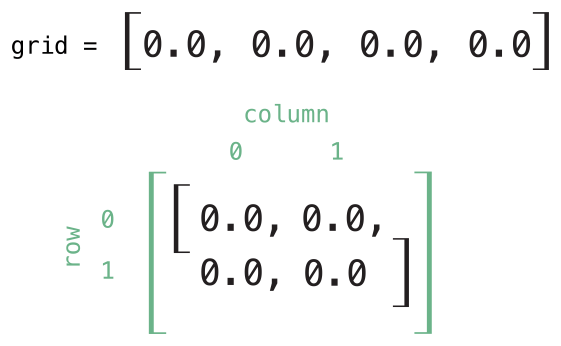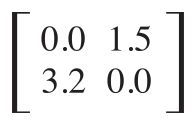# 下标脚本 - Subscripts

## 下标脚本语法

``````    subscript(index: Int) -> Int {
get {
// 返回与入参匹配的Int类型的值
}
set(newValue) {
// 执行赋值操作
}
}``````

`newValue`的类型必须和下标脚本定义的返回类型相同。与计算型属性相同的是set的入参声明`newValue`就算不写，在set代码块中依然可以使用默认的`newValue`这个变量来访问新赋的值。

``````    subscript(index: Int) -> Int {
// 返回与入参匹配的Int类型的值
}``````

``````    struct TimesTable {
let multiplier: Int
subscript(index: Int) -> Int {
return multiplier * index
}
}
let threeTimesTable = TimesTable(multiplier: 3)
println("3的6倍是\(threeTimesTable)")
// 输出 "3的6倍是18"``````

`TimesTable`例子是基于一个固定的数学公式。它并不适合开放写权限来对`threeTimesTable[someIndex]`进行赋值操作，这也是为什么附属脚本只定义为只读的原因。

## 下标脚本用法

``````    var numberOfLegs = ["spider": 8, "ant": 6, "cat": 4]
numberOfLegs["bird"] = 2``````

Swift 中字典的附属脚本实现中，在`get`部分返回值是`Int?`，上例中的`numberOfLegs`字典通过附属脚本返回的是一个`Int?`或者说“可选的int”，不是每个字典的索引都能得到一个整型值，对于没有设过值的索引的访问返回的结果就是`nil`；同样想要从字典实例中删除某个索引下的值也只需要给这个索引赋值为`nil`即可。

## 下标脚本选项

``````    struct Matrix {
let rows: Int, columns: Int
var grid: [Double]
init(rows: Int, columns: Int) {
self.rows = rows
self.columns = columns
grid = Array(count: rows * columns, repeatedValue: 0.0)
}
func indexIsValidForRow(row: Int, column: Int) -> Bool {
return row >= 0 && row < rows && column >= 0 && column < columns
}
subscript(row: Int, column: Int) -> Double {
get {
assert(indexIsValidForRow(row, column: column), "Index out of range")
return grid[(row * columns) + column]
}
set {
assert(indexIsValidForRow(row, column: column), "Index out of range")
grid[(row * columns) + column] = newValue
}
}
}``````

`Matrix`提供了一个两个入参的构造方法，入参分别是`rows``columns`，创建了一个足够容纳`rows * columns`个数的`Double`类型数组。为了存储，将数组的大小和数组每个元素初始值0.0，都传入数组的构造方法中来创建一个正确大小的新数组。关于数组的构造方法和析构方法请参考创建并且构造一个数组

``    var matrix = Matrix(rows: 2, columns: 2)````````    matrix[0, 1] = 1.5
matrix[1, 0] = 3.2```````Matrix`下标脚本的`getter``setter`中同时调用了下标脚本入参的`row``column`是否有效的判断。为了方便进行断言，`Matrix`包含了一个名为`indexIsValid`的成员方法，用来确认入参的`row``column`值是否会造成数组越界：

``````    func indexIsValidForRow(row: Int, column: Int) -> Bool {
return row >= 0 && row < rows && column >= 0 && column < columns
}``````

``````    let someValue = matrix[2, 2]
// 断言将会触发，因为 [2, 2] 已经超过了matrix的最大长度``````

App下载